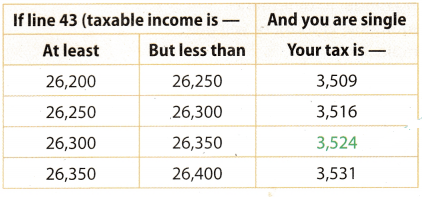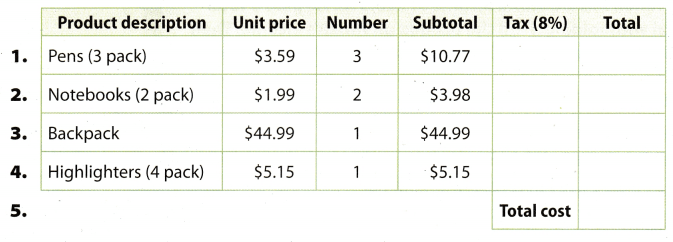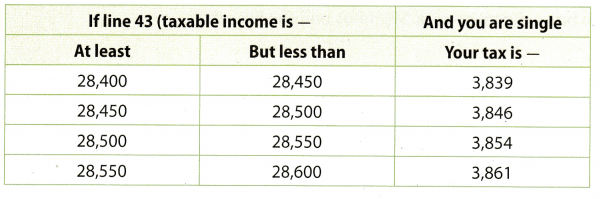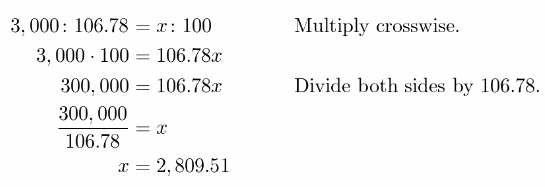# Texas Go Math Grade 7 Lesson 13.1 Answer Key Calculating Sales and Income Tax

Refer to our Texas Go Math Grade 7 Answer Key Pdf to score good marks in the exams. Test yourself by practicing the problems from Texas Go Math Grade 7 Lesson 13.1 Answer Key Calculating Sales and Income Tax.

## Texas Go Math Grade 7 Lesson 13.2 Answer Key Calculating Sales and Income Tax

Example 1.
Rafiq purchases products from a nursery for his yard. The table shows his purchases and the prices. The sales tax rate is 7.5%. Find the total cost of his purchase, including tax.
Find the tax on each item.
Black-Eyed Susan: ($6.25 . 2) . 7.5%$12.50 . 0.075 = 0.9375 = 0.94

Step 2
Add the subtotal and the tax on each item to find its total price. Add to find the total cost of his purchase.
$12.50 + 0.94 =$13.44The total cost of his purchase is $83.25. Your Turn Question 1. What If? Suppose Rafiq returned to the nursery and bought a second tree priced at$49.99 and two packages of fertilizer priced at $12.99 each. Find the total cost of his purchase, including tax. ____ Answer: Calculate tax for every product. Second tree:($49.99. 1) . 7.5%
$49.99 . 0.075 =$3.74925 = $3.75 Package of fertilizer:($12.99 . 2) . 7.5%
$25.98 . 0.075 =$1.9485 = $1.94 Find a sum for the subtotal and the tax on each of them. Total for second tree:$49.99 + $3.75 =$53.74
Total for packages of fertilizer:
$25.98 +$1.94 = $27.92Total cost of his purchase is sum of total for every product and that is$164.91.

Tax for second tree is $3.75. Tax for packages of fertilizer is$1.94 Total, cost of his purchase is $164 Example 2. Quinn’s gross monthly pay is$2,050. Federal withholding is 11.4% of her gross pay. Part of her monthly pay statement is shown in Step 2 below. Find Quinn’s net pay after all deductions.
Step 1
Find the amount that is deducted for federal withholding.
Federal withholding = $2,050 × 0.114 =$233.70
Step 2
Find the total deductions
Gross Pay
Monthly Wages $2,050.00 Taxes Federal Withholding$233.70
Social Security (6.2%) $127.10 Medicare (1.45%)$29.73
Total deductions = $233.70 +$127.10 + $29.73 =$390.53
net pay = gross pay – total deductions
= $2,050.00 –$390.53 = $1,659.47 Quinn’s net pay each month is$1,659.47.

Question 2.
Arnetta’s monthly gross pay is $4,200. Federal withholding is 16.05% of her pay. Her other deductions total$321.30. Find her net pay.
Fid the federal withholding of lier pay.
Write the percent as decimal, ie 16.05% = 0.1605.
Federal wititholding $4. 200 × 0.1605 =$674.1
To find the total deductions, add federal withholding and her other deductions.
Total deductions = $674.1 +$321.30 = $995.4 Net pay is gross pay less total deductions. Net pay = gross pay total deductions =$4,200 – $995.4 =$3,204.6
Arnetta’s net pay each month is $3,204.6. Example 3. After deductions, Jonah has a taxable income of$26,318. He paid a total of $4,059 in federal withholding. Find the tax due on Jonah’s income. Then tell whether he will have to pay an additional amount or will get a refund. Answer: Step 1 Use a tax table to find the tax for a gross income of$26,318.Step 2
Compare the tax due of $3,524 with the tax withheld of$4,059. $4,059 –$3,524 = $535 Jonah paid$535 more than he owes.

Question 3.
Glenda’s taxable income is $26,222. She paid$2,640 in federal withholding. Find the tax due on Glenda’s income. Then tell whether she will have to pay an additional amount or will get a refund. Use the tax table in Example 3.
From the table, the tax for a gross income of $26,222 is$3, 509.
She paid $2,610 in the federal withholding, which is less than the tax from the tax table. Hence, she will have to pay the difference between the tax from the table and the amount she paid in the federal withholding, which is$3,509 – $2,610 =$869
she will have to pay $869 Texas Go Math Grade 7 Lesson 13.1 Guided Practice Answer Key Find each amount. Use a sales tax rate of 8%. (Example 1) Question 1.  sno Product description Unit price Number Subtotal Tax(8%) Total 1. Pens(3 pack)$3.59 3 $10.77 Answer: Write the tax rate as decimal. ie 8% = 0.08 Tax = Subtotal × Tax Tax =$10.77 × 0.08 = $0.86 Now, add the subtotal and the tax to find the total price. Total =$10.77 + $0.86 =$11.63
Total is $11.63. The tax is$0.86.
The total is $11.63 Question 2.  sno Product description Unit price Number Subtotal Tax(8%) Total 2. Notebooks(2 pack)$1.99 2 $3.98 Answer: Write the tax rate as decimal. ie 8% = 0.08 Tax = Subtotal × Tax Tax =$3.98 × 0.08 = $0.32 Now, add the subtotal and the tax to find the total price. Total =$3.98 + $0.32 =$4.3
Total is $4.3 The tax is$0.32.
The total is $4.3 Question 3.  sno Product description Unit price Number Subtotal Tax(8%) Total 3. Backpack$44.99 1 $44.99 Answer: Write the tax rate as decimal. ie 8% = 0.08 Tax = Subtotal × Tax Tax =$41.99 × 0.08 = $3.62 Now, add the subtotal and the tax to find the total price. Total =$44.99 + $3.6 =$48.59
Total is $48.59 The tax is$3.62.
The total is $48.59. Question 4.  sno Product description Unit price Number Subtotal Tax(8%) Total 4. Highlighters(4 pack)$5.15 1 $5.15 Answer: Write the tax rate as decimal. ie 8% = 0.08 Tax = Subtotal × Tax Tax =$5.15 × 0.08 = $0.41 Now, add the subtotal and the tax to find the total price. Total =$5.15 + $0.41 =$5.56
Total is $5.56 The tax is$0.41.
The total is $5.56. Question 5.Answer: To find the total cost, add all totals which we calculated in the previous questions. Total cost =$11.63 + $4.3 +$48.59 + $5.56 =$70.08
The total cost is $70.08. Question 6. Juan earns a monthly salary of$3,200. Federal withholding is 14.1% of his gross pay. Juan has a total of $244.80 deducted for Social Security and Medicare. Find his net pay. (Example 2) Answer: First, find the federal withholding of his pay. Write the percent as decimal, ie 14.1% = 0.141. Federal withholding =$3,200 × 0.141 = $451.2 To find the total deductions, add federal withholding and deductions for Social Security and Medicare. Total deductions =$451.2 + $244.80 =$696
Net pay is gross pay less total deductions.
Net pay = gross pay — total deductions
= $3, 200 –$696 = $2. 501 Juan’s net pay each month is$2, 504.

Question 7.
Lizaveta’s taxable income is $26,380. She paid$2,940 in federal withholding. Use a problem solving model to determine whether she will have to pay an additional amount or will get a refund. Use the tax table in Example 3. (Example 3)
From the table, the tax for a gross income of $26,380 is$3,531.
She paid $2,940 in the federal withholding, which is less than the tax from the tax table. Hence, she will have to pay the difference between the tax from the table and the amount she paid in the federal withholding. which is$3,531 – $2,910 =$591
She will have to pay $591. Essential Question Check-In Question 8. Suppose the sales tax rate in your area is 7.5%. Explain how to determine the total cost, including tax, of an eReader that costs$125.
First, we have to find the tax of an eReader.
Write percent as decimal, ie 7.5% = 0.075.
Multiply the cost of eReader by the sales tax rate to find the find the tax.
$125 × 0.075 =$9.375
The tax of an eReader is $9.375. To find the total cost. of eReader add the cost of eReader and the tax.$125 + $9.375 =$134.375
The total cost of eReader is $134.375. Texas Go Math Grade 7 Lesson 13.1 Independent Practice Answer Key Question 9. Roland is purchasing pottery supplies for his pottery class. He buys a portable pottery wheel for$382.00, modeling clay for $12.00, and a pottery tool set for$16.14. If the tax rate is 8.25%, find the total tax he paid. Then find the total cost of his purchases.
First, we have to find the tax of each item.
The tax rate is 8.25%.
Write the tax rate as decimal, ie 8.25% = 0.0825.
A portable pottery wheel priced at $38200 Tax =$382.00 × 0.0825 = $31.515 Now, add the total and the tax to find the total price. Total =$382.00 + $31.515 =$413.515
Modeling clay priced at $12.00 Tax =$12.00 . 0.0825 = $0.99 Now, add the total and the tax to find the total price. Total =$12.00 + $0.99 =$12.99
Pottery tool set priced at $16.14 Tax =$16.14 . 0.0825 = $1.33 Now, add the total and the tax to find the total price. Total =$16.14 + $1.33 =$17.47
To find the total tax he paid, totalize all taxes.
Total Tax = $31.515 +$0.99 + $1.33 =$33.835
Finally, add all total prices to find the total cost of his purchase.
$413.515 +$12.99 + $17.47 =$443.975
The total cost of his purchase is $443.975. The total tax he paid is$33.835.
The total cost of his purchase is $443.975. Question 10. Megna has$300 to buy a digital camera. She finds one she likes that costs $275. a. Suppose the tax rate is 7.3%. Does Megna have enough to buy the camera? Explain. Answer: First. find the tax of a digital camera that costs 8275. Vrite the tax rate as decimal. ie 7.3% = 0.073. Tax =$275 × 0.073 = $20.075 Now, add the cost of a digital camera and the tax to find to total cost of a digital camera. Total cost =$273 + $20.075 =$295.073
The total cost of a digital camera that Megna wants to buy is $295.075, hence she has enough money to buy it. b. Megna discovers that the digital camera needs a memory card. She finds a memory card on sale for$9.99 plus tax. Can Megna afford the card?
The total cost of a digital camera is $295.075 and Megna has$300.
After she buys a digital camera, she remains $4.925. A memory card costs$9.99 plus tax, which is more from what she had left.
Hence, she can not afford the memory card.

Question 11.
Casey is looking at the used car shown. Suppose that Casey is able to get $1,200 in trade for his old vehicle. The sales tax rate is 7.75%. How much will Casey pay in taxes on the reduced sales price? Answer: Determine the price of the used car when Casey traded his old vehicle. 12,999 – 1,200 = 11,799 After the trade, the car cost$11,799. Determine the sales tax for the car.
Show the tax rate as a decimal.
11.799 × 0.0775 = 914.4225 ≈ 914.42
The sales tax for the car is $914.42. Question 12. What’s the Error? Lauren wants to buy 3 shirts at$15.00 each. At home, she calculates the totai cost as $46.20, using the sales tax rate of 8%. At the store, the cashier tells Lauren the total cost is$48.60. Who is wrong and what mistake did he or she make?
Each shirt costs $15.00 and 3 shirts cost 3 ×$15.00 = $45. Write the tax rate as decimal. ie 8% = 0.08. First, find the tax of 3 shuts. Tax =$45 × 0.08 = $3.6 Now, add the cost of 3 shirts and the tax to find the total cost of shirts. Total cost =$45 + $3.6 =$18.6
The total cost of 3 shirts is $48.6, hence Lauren made a mistake. The mistake Lauren made is that she did not calculated the tax of all 3 shirts. but only of one shirt. Let ‘s check. The tax of one shirt is The tax =$15 × 0.08 = $1.2 Now, add the cost of 3 shirts mid the tax of one shirt to find the total cost. Total cost =$45 + $1.2 =$46.2

Question 13.
Camden earns $25 per hour, and works 40 hours a week. a. Camden gets paid every 2 weeks. Federal withholding is 15.6% of his gross pay, Social Security is 6.2% of his gross pay, and Medicare is 1.45%. Find Camden’s net pay after 2 weeks. Show your work. Answer: First, find Camden’s gross pay for 2 weeks. He works 40 hours a week, hence he works 80 hours for 2 weeks. He earns$25 per hour. hence lie earns 80 × $25 =$2, 000 for 2 weeks.
First, find the Federal withholding of his gross pay.
Write the percent as decimal, ie 15.6% = 0.156.
Federal withholding = $2,000 × 0.156 =$312
Second, find the Social Security of his gross pay.
Write the percent as decimal, ie 6.2% = 0.062.
Social Security = $2. 000 × 0.062 =$124

Third, find the Medicare of his gross pay.
Write the percent as decimal, ie 1.45% = 0.0145.
Social Security = $2,000 × 0.0145 =$29
To find the total deductions, add Federal withholding and Social Security and Medicare.
Total deductions = $312 +$124 + $29 =$465
Net pay is gross pay less total deductions.
Net pay = gross pay – total deductions
= $2,000 –$465 = $1,535 Camden’s net pay after 2 weeks is$1,535.

b. What If suppose Camden gets a raise of $5 per hour and pays 17.2% of his gross pay in federal withholding. What would his net pay for 2 weeks be? Make sure to factor in his social security and Medicare withholdings. Answer: Now, suppose Camden gets a raise of$5 per hour, hence he earns $30 per hour. He works 80 hours for 2 weeks, so he earns 80 ×$30 = $2,400 for 2 weeks. Also, Federal withholding is now 17.2%. Find the Federal withholding of his gross pay. Write the percent as decimal, ie 17.2% = 0.172. Federal withholding =$2, 400 × 0.172 = $412.8 The rate of Social Security and Medicare are the same as in Part a. Find the Social Security of his gross pay. Write the percent as decimal, ie 6.2% = 0.062. Social Security =$2. 100 × 0.062 = $148.8 Find the Medicare of his gross pay. Write the percent as decimal, ie 1.45% = 0.0145. Medicare =$2. 400 × 0.0 145 = $34.8 To find the total deductions. add Federal withholding and Social Security and Medicare. Total deductions =$412.8 + $148.8 +$34.8 = $596.4 Net pay is gross pay less total deductions. Net pay = gross pay – total deductions =$2,400 – $569.4 =$1,803.6
Camden’s net pay after 2 weeks will be $1, 803.6. Question 14. Critique Reasoning Samantha said adding 6% to the cost of an item for sales tax makes the total cost 106% of the original price. Joshua disagrees. Is Samantha’s reasoning accurate? Explain. Answer: Yes, it is. When you calculate the sales tax of an item, you calculate it of the original, price of that item, and that original price represents the 100% of that item. So, when you calculate 6% of the original price and add it to the original price, which is 100%, that sum is 100% + 6% = 106% of the original price. Question 15. After deductions, Elizabeth’s taxable income was$28,536. She had $344 withheld each month for taxes. Use the tax table shown to find how much she will get as a refund or owe.Answer: From the table, the tax for taxable income of$28,536 is $3,854. She had$341 withheld each month for taxes, so for a year she had withheld
12 × $344 =$4,128
The amount she had withheld is greater than the amount of tax is. so she will get a refund.
The refund = $4. 128 –$3,854 = $274 She will get a refund of$274.

Texas Go Math Grade 7 Lesson 13.1 H.O.T. Focus on Higher Order Thinking Answer Key

Question 16.
Persevere in Problem Solving A family buys groceries for $149.98, including$10.07 in taxes. The bill includes $26.95 worth of items that are not taxable. Find the sales tax rate. Round your answer to the nearest tenth of a percent. Answer: First, we have to subtract the worth of items that are not taxable from the whole bill, to find the bill of all groceries that are taxable.$149.98 – $26.95 =$123.03
$123.03 is the bill of all groceries that are taxable. Now, taxes apply only to this amount,$123.03.
We have to find how much $10.07 is actually a percentage of$123.03 and that would represent the sales tax rate.
How to find what percent of X is Y.
The percentage formula is
$$\frac{Y}{X}$$ = P%
In our case, Y = 10.07 and X = 123.03.
$$\frac{10.07}{123.03}$$ = 0.08
The result will always be in decimal form, not percentage form.
We need to multiply the result by 100 to get the percentage.
0.08 × 100 = 8
Hence, the sales tax rate is 8%.

Question 17.
Multistep Suppose the personal income tax rate is changed to a flat rate of 15% of one’s taxable income. Use the tax table in problem 15 to investigate the effect of the change in the rate on a single taxpayer with a taxable income of $28,443. Answer: From the table, the tax for taxable income of$28,113 is $3,839. Let’s find how much$3,839 is actually a percentage of $28,413 and that would represent the tax rate from the table. How to find what percent of X is Y. The percentage formula is $$\frac{Y}{X}$$ = P% In our case, Y = 3,839 and X = 28,443. $$\frac{3,839}{28,443}$$ = 0.13 The result will always be in decimal form, not percentage form. We need to multiply the result by 100 to get the percentage. 0.13 × 100 = 13 The tax rate from the table is 13%. which is less than 15%. Hence, this change would effect bad on a single taxpayer because lie would pay more if the tax rate is 15%. This change would effect bad on a single taxpayer because he would pay more if the tax rate is 15%. Question 18. Represent Real-World Problems You have$3,000 to spend on a laptop. Suppose the tax rate is 6.78%. What is the maximum you can spend on a laptop if you know you will also be purchasing a $25 case? Explain. Answer: When you add the tax rate on the cost of some item, you get the total cost of that item. Total cost = The cost + The tax The cost of a item represents 100% of that item and when you add the tax rate(given in percent), the total cost in percent will be Total cost% = 100% + Tax rate(%) In this case, you have$3,000 to spend on a laptop and a case.
Hence, the total cost of a laptop and a case must not be greater than $3.000. The tax rate is 6.78%. so the total cost of a laptop and a case in percent is 100% + 6.78% = 106.78%$3,000 is actually 106.78%. and we want to know how much money is 100% which is, in our case, the cost of a laptop and a case.
We can set a proportion to calculate that.
$3,000 represents 106.78%, and we want to know how much money is 100%. Let x be the amount of money that represents this 100%, ie the maximum amount of money we can spend on a laptop and a case. The proportion:The cost of a laptop and a case is$2, 809.51.
We know that the cost of a case is $25. so we have to subtract the cost of a case from$2, 809.51.
$2, 809.51 –$25 = $2,781.51 The maximum cost of a laptop is$2,784.51.

Scroll to Top